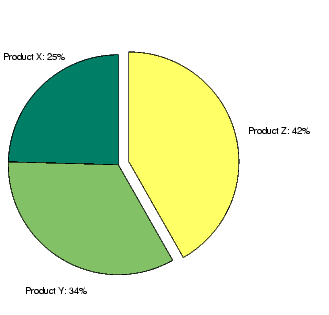GraphicsPie Charts

Pie charts display the percentage that each element in a vector or matrix contributes to the sum of all elements. `pie` and `pie3` create 2-D and 3-D pie charts.

Example -- Pie Chart

Here is an example using the `pie` function to visualize the contribution that three products make to total sales. Given a matrix `X` where each column of `X` contains yearly sales figures for a specific product over a five-year period,

• ``````X = [19.3 22.1 51.6;
``````     34.2 70.3 82.4;
``````     61.4 82.9 90.8;
``````     50.5 54.9 59.1;
``````     29.4 36.3 47.0];
``````

sum each row in `X` to calculate total sales for each product over the five-year period.

• ``````x = sum(X);
``````

You can offset the slice of the pie that makes the greatest contribution using the `explode` input argument. This argument is a vector of zero and nonzero values. Nonzero values offset the respective slice from the chart.

First, create a vector containing zeros.

• ```explode = zeros(size(x));
```

Then find the slice that contributes the most and set the corresponding `explode` element to `1`.

• ```[c,offset] = max(x);
explode(offset) = 1;
```

The `explode` vector contains the elements `[0 0 1]`. To create the exploded pie chart, use the statement

• ```h = pie(x,explode); colormap summer```

Labeling the Graph

The pie chart's labels are text graphics objects. To modify the text strings and their positions, first get the objects' strings and extents. Braces around a property name ensure that `get` outputs a cell array, which is important when working with multiple objects.

• ```textObjs = findobj(h,'Type','text');
oldStr = get(textObjs,{'String'});
val = get(textObjs,{'Extent'});
oldExt = cat(1,val{:});
```

Create the new strings, then set the text objects' `String` properties to the new strings.

• ``````Names = {'Product X: ';'Product Y: ';'Product Z: '};
```newStr = strcat(Names,oldStr);
set(textObjs,{'String'},newStr)
```

Find the difference between the widths of the new and old text strings and change the values of the `Position` properties.

• ```val1 = get(textObjs, {'Extent'});
newExt = cat(1, val1{:});
```offset = sign(oldExt(:,1)).*(newExt(:,3)-oldExt(:,3))/2;
```pos = get(textObjs, {'Position'});
textPos =  cat(1, pos{:});
textPos(:,1) = textPos(:,1)+offset;
set(textObjs,{'Position'},num2cell(textPos,[3,2]))
```Comparing Data Sets with Area Graphs Removing a Piece from a Pie Chart© 1994-2005 The MathWorks, Inc.